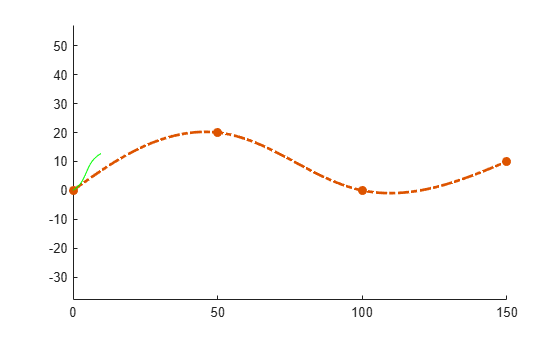# referencePathFrenet

Smooth reference path fit to waypoints

Since R2020b

## Description

The `referencePathFrenet` object fits a smooth, piecewise, continuous curve to a set of waypoints given as ```[x y]``` or ```[x y theta]```. After fitting, points along the curve, the path points are expressed as ```[x y theta kappa dkappa s]```, where:

• x y and theta— SE(2) state expressed in global coordinates, with x and y in meters and theta in radians

• kappa — Curvature, or inverse of the radius, in `m-1`

• dkappa — Derivative of curvature with respect to arc length in `m-2`

• s — Arc length, or distance along path from path origin, in meters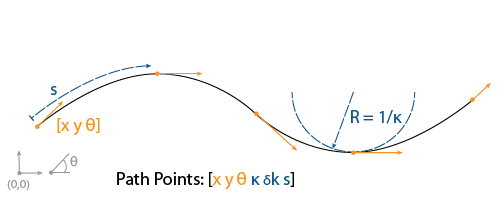Using this object, convert trajectories between global and Frenet coordinate systems, interpolate states along the path based on arc length, and query the closest point on a path from a global state.

The object expresses Frenet states as a vector of form ```[S dS ddS L dL ddL]```, where S is the arc length and L is the perpendicular deviation from the direction of the reference path. Derivatives of S are relative to time. Derivatives of L are relative to the arc length, S.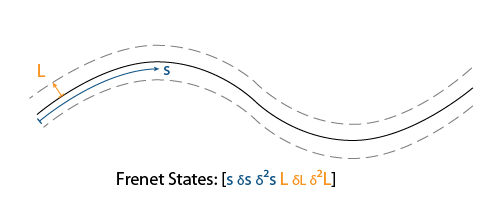## Creation

### Syntax

``refPathObj = referencePathFrenet(waypoints)``
``refPathObj = referencePathFrenet(___,Name,Value)``

### Description

example

````refPathObj = referencePathFrenet(waypoints)` fits a piecewise, continuous set of curves to the specified waypoints. The `waypoints` argument sets the `Waypoints` property.```
````refPathObj = referencePathFrenet(___,Name,Value)` sets properties using one or more name-value pair arguments in addition to the input arguments in the previous syntax. You can specify the DiscretizationDistance and MaxNumWaypoints properties as name-value arguments.```

## Properties

expand all

Presampled points along the path, specified as a P-by-2 matrix with rows of form ```[x y]``` or P-by-3 matrix with rows of form ```[x y theta]```. Specify x and y in meters and theta in radians. P is the number of presampled points, and must be greater than or equal to two.

Data Types: `single` | `double`

Total arclength along the path in meters.

Data Types: `single` | `double`

Clothoid parameters at start of segments, specified as an N-by-6 matrix where N is the total number of waypoints. Each row contains the parameters of the clothoidal segment to connect to the next consecutive waypoint and is in the form ```[x y theta kappa dkappa s]``` where:

• x y and theta — SE(2) state expressed in global coordinates, with x and y in meters and theta in radians

• kappa — Curvature, or inverse of the radius, in `m-1`

• dkappa — Derivative of curvature with respect to arc length in `m-2`

• s — Arc length, or distance along path from path origin, in meters

Data Types: `single` | `double`

Arc length between interpolated path points, specified as a positive scalar in meters. The object uses interpolated path points to accelerate performance of the transformation functions `frenet2global` and `global2frenet`. A smaller discretization distance can improve accuracy at the expense of memory and computational efficiency.

Example: ```refPath = referencePathFrenet(waypoints,'DiscretizationDistance',0.4)```

Data Types: `single` | `double`

Maximum number of waypoints allowed in the path, specified as either `Inf` for resizeable path or a positive integer to enforce a static limit.

If `MaxNumWaypoints` is set to `Inf`, to generate code, `DynamicMemoryAllocation` must be set to `'on'`.

Example: ```refPath = referencePathFrenet(waypoints,'MaxNumWaypoints',10)```

Data Types: `single` | `double`

## Object Functions

 `closestPoint` Find closest point on reference path to global point `closestPointsToSequence` Projects sequence of points onto path `closestProjections` Find orthogonal projections between path tangent vector and query point `curvature` Return curvature at arclength `changeInCurvature` Return change-in-curvature at arclength `frenet2global` Convert Frenet states to global states `global2frenet` Convert global states to Frenet states `interpolate` Interpolate reference path at provided arc lengths `position` Return xy-position at arclength `tangentAngle` Return tangent angle at arclength `show` Display reference path in figure `copy` Copy reference path

## Examples

collapse all

Generate alternative trajectories for a reference path using Frenet coordinates. Specify different initial and terminal states for your trajectories. Tune your states based on the generated trajectories.

Generate a reference path from a set of waypoints. Create a `trajectoryGeneratorFrenet` object from the reference path.

```waypoints = [0 0; ... 50 20; ... 100 0; ... 150 10]; refPath = referencePathFrenet(waypoints); connector = trajectoryGeneratorFrenet(refPath);```

Generate a five-second trajectory between the path origin and a point 30 m down the path as Frenet states.

```initState = [0 0 0 0 0 0]; % [S ds ddS L dL ddL] termState = [30 0 0 0 0 0]; % [S ds ddS L dL ddL] [~,trajGlobal] = connect(connector,initState,termState,5);```

Display the trajectory in global coordinates.

```show(refPath); hold on axis equal plot(trajGlobal.Trajectory(:,1),trajGlobal.Trajectory(:,2),'b') legend(["Waypoints","Reference Path","Trajectory to 30m"])```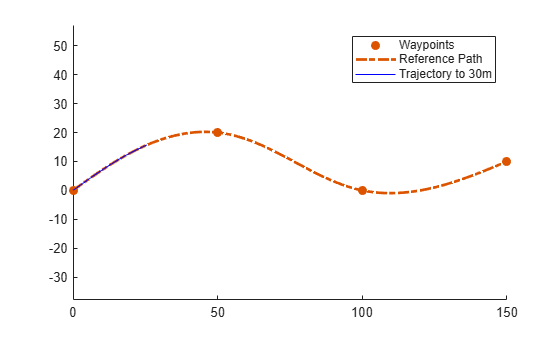Create a matrix of terminal states with lateral deviations between –3 m and 3 m. Generate trajectories that cover the same arc length in 10 seconds, but deviate laterally from the reference path. Display the new alternative paths.

```termStateDeviated = termState + ([-3:3]' * [0 0 0 1 0 0]); [~,trajGlobal] = connect(connector,initState,termStateDeviated,10); clf show(refPath); hold on axis equal for i = 1:length(trajGlobal) plot(trajGlobal(i).Trajectory(:,1),trajGlobal(i).Trajectory(:,2),'g') end legend(["Waypoints","Reference Path","Alternative Trajectories"]) hold off```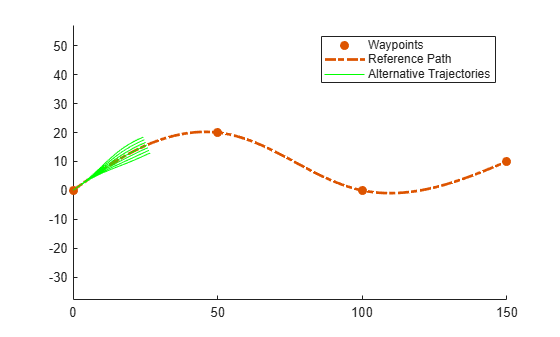Specify a new terminal state to generate a new trajectory. This trajectory is not desirable because it requires reverse motion to achieve a longitudinal velocity of 10 m/s.

```newTermState = [5 10 0 5 0 0]; [~,newTrajGlobal] = connect(connector,initState,newTermState,3); clf show(refPath); hold on axis equal plot(newTrajGlobal.Trajectory(:,1),newTrajGlobal.Trajectory(:,2),'b'); legend(["Waypoint","Reference Path","New Trajectory"]) hold off```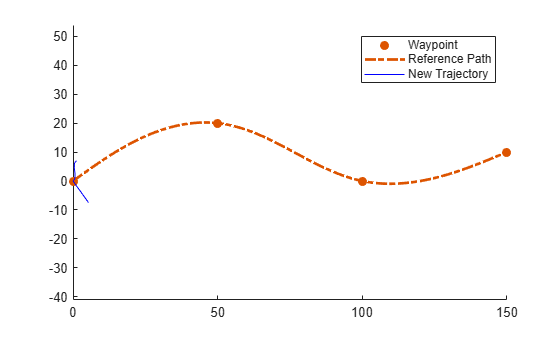Relax the restriction on the longitudinal state by specifying an arc length of `NaN`. Generate and display the trajectory again. The new position shows a good alternative trajectory that deviates off the reference path.

```relaxedTermState = [NaN 10 0 5 0 0]; [~,trajGlobalRelaxed] = connect(connector,initState,relaxedTermState,3); clf show(refPath); hold on axis equal plot(trajGlobalRelaxed.Trajectory(:,1),trajGlobalRelaxed.Trajectory(:,2),'g'); hold off```